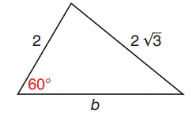Chapter 11.4, Problem 36E### Elementary Geometry for College St...

6th Edition
Daniel C. Alexander + 1 other
ISBN: 9781285195698

#### Solutions

Chapter
Section### Elementary Geometry for College St...

6th Edition
Daniel C. Alexander + 1 other
ISBN: 9781285195698
Textbook Problem
1 views

# For the triangle shown, use the Law of Cosines to determine b.To determine

To find:

The length b of the given triangle by using the law of cosines.

Explanation

Formula:

The Law of Cosines

a2=b2+c22bccosα

b2=a2+c22accosβ

c2=a2+b22abcosγ

Where α, β, and γ is the acute angle of the triangle, and a, b, and c is the sides of the triangle.

Calculation:

Given,

From the triangle,

Take a=2,c=23,andγ=60

By the law of cosines,

c2=a2+b22abcosγ

(23)2=(2)2+b22(2)bcos(60)

Since, cos60=12

4(3)=4+b2

### Still sussing out bartleby?

Check out a sample textbook solution.

See a sample solution

#### The Solution to Your Study Problems

Bartleby provides explanations to thousands of textbook problems written by our experts, many with advanced degrees!

Get Started

#### Expand each expression in Exercises 122. x(3x+y)

Finite Mathematics and Applied Calculus (MindTap Course List)

#### Find the limit: limx2x38x2.

Calculus: An Applied Approach (MindTap Course List)

#### I=ER,E=120,andR=12.findI.

Elementary Technical Mathematics

#### The slope of the tangent line to y = x3 at x = 2 is: 18 12 6 0

Study Guide for Stewart's Single Variable Calculus: Early Transcendentals, 8th